# Length - math word problems

1. Cuboid - volume, diagonalsThe length of the one base edge of cuboid a is 3 cm. Body diagonal is ut=13 cm and diagonal of cuboid's baseis u1=5 cm. What is the volume of the cuboid?
2. Bar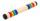Half of the bar is green, the third is yellow and the remaining 40cm is red. How long is a bar?
3. Prism - boxThe base of prism is a rectangle with a side of 7.5 cm and 12.5 cm diagonal. The volume of the prism is V = 0.9 dm3. Calculate the surface of the prism.
4. Cyclist and carA cyclist who runs at a speed of 20km per hour will arrive at the finish in 4.5 hours. How fast does the car have to drive the same route in 2 hours?
5. Square tilesThe room has dimensions 12 meters and 5.6 meters. Determine the number of square tiles and their largest dimension to exactly cover the floor.
6. CostumeDenisa is preparing for a goldsmith's costume carnival. During the preparations, she thought she would let her hair wipe instead - she would apply a 5 μm thick layer of gold to each hair. How much gold would Denisa need? Assume that all hundred thousand D
7. The bookThe book has 280 pages. Each side is a rectangle with sides 15 cm and 22 cm. a) how many leaves has the book? b) at least how many square meters of paper should be used to production this book?
8. Bridge piers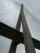One quarter of the bridge pier is sunk into the ground. Two thirds are in the water. Protruding above the water is 1.20 m long. Determine the height of bridge piers.
9. Average height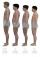In a class are 34 students. The average height of students is 165 cm. What will be the average height of students in the classroom when two pupils tall 176 cm and 170 cm moved from this school/class?
10. Train expres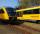At 8.00 strarted from Bratislava to Poprad express Tatry. Cities are distant 340 kilometers. At the same time started from Poprad express Danube to Bratislava. Express Tatry's average speed is 80 km/h, Danube 90 km/h. At what distance from Poprad trains
11. CloudsFrom two points A and B on the horizontal plane was observed forehead cloud above the two points under elevation angle 73°20' and 64°40'. Points A , B are separated by 2830 m. How high is the cloud?
12. Tablecloth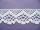The table top is 90 cm long and 50 cm wide. How many cm lace edging is necessary for the tablecloth that hangs on each side by 10 cm?
13. Ascent and descent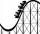Car goes 114 km track, which consists of ascent and descent at time of 1 hour 35 minutes. When climbing moves at the speed 48 km/h and downhill 25 m/s. What is the length of climb and descent?
14. Trapezium ABCDIn the figure, ABDC is a trapezium in which AB || CD. line segments RN and LM are drawn parallel to AB such that AJ=JK=KP. If AB=0.5m and AP=BQ=1.8m, find the lengths of AC, BD, RN and LM. angle D=angle C=60
15. Three drivers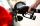Three driversdriving the same direction found that they have same amounth of gasoline. The first is enough to go 6 km, 4 km second and third 3km. Gasoline they divided so all three just drove to the nearest petrol station. How many km away was a petrol st
16. Wood cuboidWhat is the weight of the wood cuboid 15 cm, 20 cm, 3 m if 1 m3 wood weighs 800 kg?
17. Candelas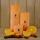We burned two unequally thick and long candles. Longer burnt for three and a half hours and shorter for five hours. After two hours of burning it was identical. How many times was longer candle longer then shorter?
18. AceThe length of segment AB is 24 cm and the point M and N divided it into thirds. Calculate the circumference and area of this shape.
19. Gasoline consumption Skoda MB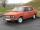Consumption of cars for 100 km is 6.6 liters of gasoline 1 liter of gasoline costs € 1.274. How many euro grandfather spent when the car travelled 321 km.
20. Arc-sectorarc length = 17 cm area of sector = 55 cm2 arc angle = ? the radius of the sector = ?

Do you have an interesting mathematical word problem that you can't solve it? Submit math problem, and we can try to solve it.

We will send a solution to your e-mail address. Solved examples are also published here. Please enter the e-mail correctly and check whether you don't have a full mailbox.

Please do not submit problems from current active competitions such as Mathematical Olympiad, correspondence seminars etc...

Do you want to convert length units?